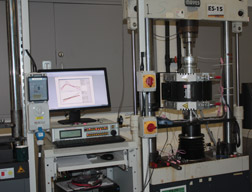# Thermo-Mechanical Fatigue (TMF)

Thermo Mechanical Fatigue (TMF) testing is mainly done, to determine the total lifetime of technical components (e. g. turbine blades of aeroplane engines (jet engines) and gas turbines) previous to the technical implementation of that part. It is a variation of mechanical fatigue of materials where heating and cooling cycles are applied to a test material additional to a mechanical cyclic loading.

The temperature cycles have - in most cases - the same frequency as the loading cycles, but have different phase shifts. If the phase shift is δ=0 the TMF tests are called in-phase tests, i. e. a tensile force is applied to the specimen while heating is done. If the phase shift is δ=180° the TMF test are called out-of-phase tests, i. e. the sample is cooled while in tension. For special testing parameters the phase shift can be applied between 360° > δ > 0, to fit the real requirements for the tested material in later application fields.

Dirlik Controls provides machine control, data acquisition and analysis for Thermo-Mechanical Fatigue (TMF) Testing. The test specimen is subjected to cyclic, externally imposed temperature and mechanical strain fields, simultaneously varied, controlled and recorded.

The specimen geometry and the set-up of the heating system are optimised with respect to a temperature field T . The mechanical strain cycle is then realized by controlling the total strain, εtot, applied to the specimen in a way that the thermal strain, εth, is compensated to achieve a given mechanical strain, εm = εtot − εth, and the response of the specimen is measured in terms of the cyclic stress σ.

Before starting a TMF test series the evaluation of E modulus in the selected temperature range is carried out at RT and every 50°C between Tmin and Tmax. The E modulus can be determined in static or pseudodynamic procedure.M

During the test, the time and cycle number, temperature, total strain and force are recorded with a minimum sampling rate of 200 points per cycle. From load, strain and temperature data, plots of stress, mechanical strain, and temperature as a function of time, and plots of mechanical strain as a function stress and of temperature can be generated.

The elastic modulus, measured as a function of temperature; the thermal strain as a function of temperature and the stress-temperature path from the zero stress shall be plotted and tabulated for each specimen.

End of TMF life is defined as the point at which the maximum stress σmax or stress range Δσ decreases by user-defined level, e.g. 10% below a tangent line drawn to the curve of σmax or Δσ versus N in linear scaling; or total separation of the test piece.